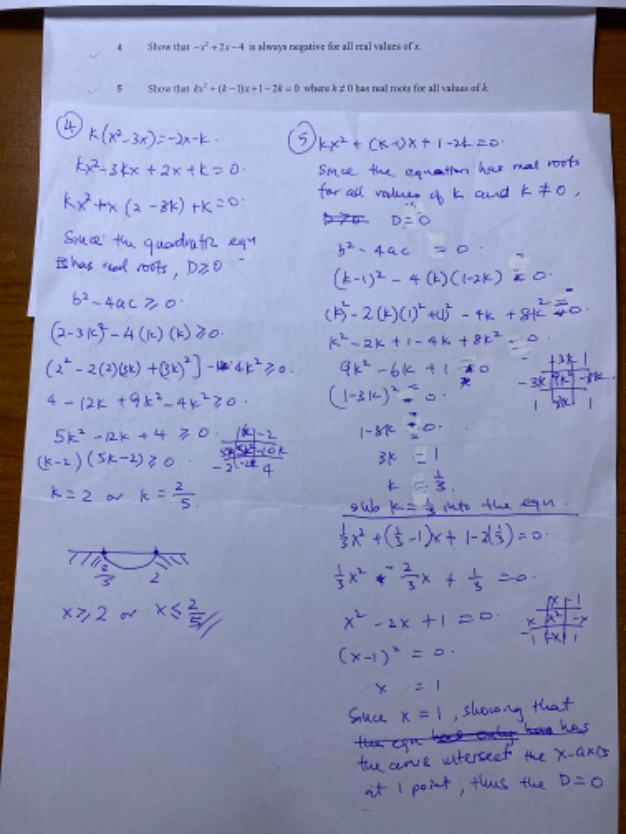# QuestionKindly help me to see whether my presentation to both questions are correct thanks

Your Question 4 solution does not match the Question 4 in your paper.

Question 4

Show that -x2 +2x – 4 is always negative for all real value of x.

In order for a quadratic function to be always negative, it must be

1.  b²-4ac < 0
2.  a < 0

for all real values of x.

a =-1, b = – 2, c = -4

a < 0.   second condition is satisfied.

b²-4ac = (-2)2 – 4(-1)(-4) = 4 – 16 = -12 < 0   first condition is satisfied

Hence -x2 +2x – 4 is always negative for all real value of x.

Question 5:

Show that kx2  + (k-1)x + (1-2k)  = 0   where k <> 0,  has real roots for all values of k.

For real rootsb²-4ac  ≥ 0

a = k, b = (k-1), c = (1-2k)

(k-1)2 – 4(k)(1-2k)

k2 – 2k + 1 – 4k +8k

9k2 -6k + 1   = (3k-1)(3k-1)

This means (3k-1)2   ≥ 0 for all values of k and that the quadratic equation has real roots.

3k ≥ 1

k ≥  (1/3)   i.e. k <> 0

So for all k ≥ (1/3) gives kx2  + (k-1)x + (1-2k)  = 0  real roots  and k <> 0.